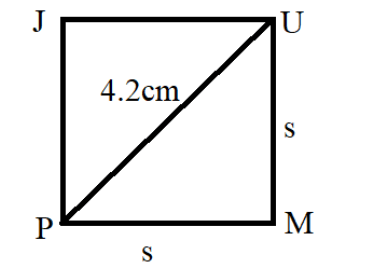Courses
Courses for Kids
Free study material
Free LIVE classes
More# Find the area of a square JUMP whose length of diagonal is $4.2\,cm$ ?

Last updated date: 15th Mar 2023
Total views: 204k
Views today: 4.83kVerified
204k+ views
Hint: Here in this question, we have to find the area of a square whose length of diagonal is given to us as $4.2\,cm$ . To find the area of a square, we have a standard formula $Area = {\left( {Side} \right)^2}$ . We can find the length of the side of the square by using the Pythagoras theorem. Then, we substitute the known values of the side of the square and determine the area of the square using the formula.

A square is a two dimensional shape with four equal sides. So, it is a quadrilateral with all of the sides being equal and each angle being a right angle.
To determine the area of a square, we have standard formula $A = {\left( {Side} \right)^2}$ where A represents the area of the square. The side of a square is the line segment which joins two consecutive vertices of a square. The side of a square is often denoted as ‘S’ or ‘s’. The unit for the area is square units.
In the given question, we are given the length of the diagonal of the square as $4.2\,cm$ .
So, we can find the length of the side of the square using the Pythagoras theorem and then get the area of square using the formula $A = {\left( {Side} \right)^2}$ in the unit $c{m^2}$ .
So, to find the length of the side of the square, we first draw a rough sketch of the square JUMP whose diagonal is 4.2 centimetres long.
So, we get,Using the Pythagoras theorem in any one of the two right angled triangles formed by constructing the diagonal of rectangle, we get,
$\Rightarrow {(Diagonal)^2} = (Side){}^2 + {(Side)^2}$
Substituting the value of the diagonal of the square and simplifying the equation, we get,
$\Rightarrow {(4.2cm)^2} = 2(Side){}^2$
$\Rightarrow {(Side)^2} = \dfrac{{{{\left( {4.2cm} \right)}^2}}}{2}$
We can find the value of the side of the square by taking the square root on both sides of the equation. But, we don’t need to take the square root as we just have to find the area of the square that can be computed by using the value of ${(Side)^2}$ .
To find the area of a square, we use formula $A = {\left( {Side} \right)^2}$ . We know the value of ${(Side)^2}$ from the equation $\left( 1 \right)$ . So, we get,
$\Rightarrow A = {\left( {Side} \right)^2} = \dfrac{{{{\left( {4.2cm} \right)}^2}}}{2}$
Square of a number can be calculated by multiplying the number with itself. So, we compute the square of the number and simplify the expression,
$\Rightarrow A = \dfrac{{17.64}}{2}c{m^2}$
$\Rightarrow A = 8.82\,c{m^2}$
Hence the area of a square whose length of diagonal is given to us as $4.2\,cm$ is $8.82\,c{m^2}$ .

Note: Generally the area is the region occupied by the thing. The area of a square is defined as the region occupied by the quadrilateral region. It can be determined by using formula $A = {s^2}$ where s represents the side of the square and A denotes the area of the square.